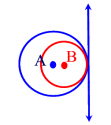Monday, September 14, 2009

Common Tangent To A Pair Of Circles

Common tangents are lines or segments that are tangent to more than one circle at the same time.

The possibility of common tangents is closely linked to the mutual position of circles.

If two circles touch inside, the two internal tangents vanish and the two external ones become a single tangent.If two circles intersect, the common tangent is replaced by a common secant, whence there are only two external tangents.If two circles touch each other outside, the two internal tangents coincide in a common tangent, thus there are three common tangents.If two circles are separate, there are four common tangents, two inside and two outside.1.2.